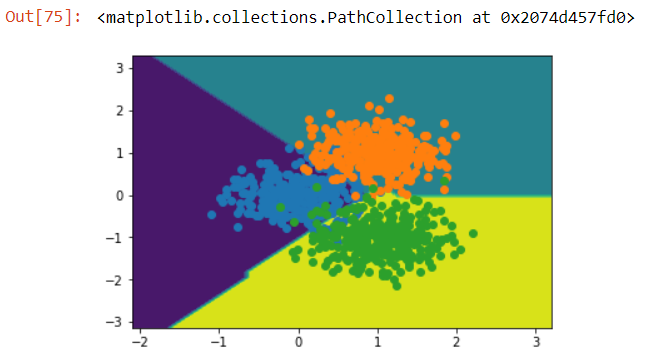# Multi-class Classification with Deep Learning

Source: Deep Learning on Medium# Multi-class Classification with Deep Learning

Hello, today I am going to do multi-class classification using Keras. The activation function that I have chosen is the Softmax function. Let’s talk about why I chose it.

The crucial difference between using binary dataset as opposed to dealing with multiclass dataset is the replacement of the activation functions such as Sigmoid. The Sigmoid function gives us the probability ranging from 0 to 1:

But now we are introducing more than 2 classes. So this function is not an ideal choice anymore. Instead we will use Softmax Function. The equation of the same is below: